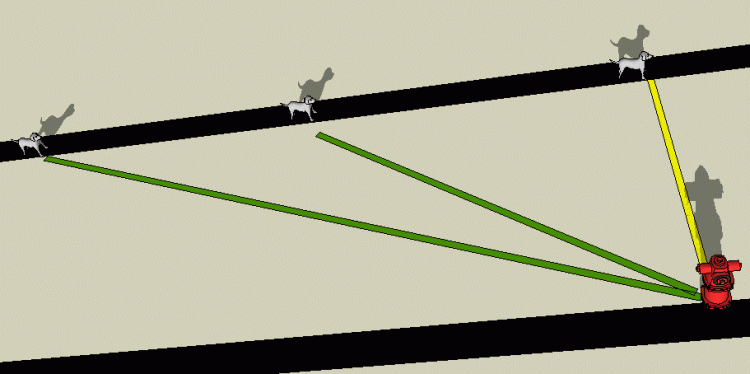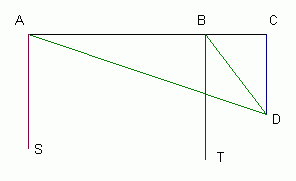SEARCH HOMEMath Central Quandaries & QueriesQuestion from Brittany, a student: A bulldog is walking east along Main St. at a speed of 4 miles per hour. He wants to get to a fire hydrant on a parallel street. Initially, the bearing to the hydrant is south 62 degrees east. After 20 minutes, the bearing is south 41 degrees east. If the bulldog continues his walk, what is the closest he will be to the hydrant?Hi Brittany. I don't have a bulldog and I won't draw this to scale, but here's the idea:Can you see the two right triangles here? We want to know the length of the yellow line (the side opposite the two angles with dogs. Here is a more geometric view:Consider the trig function Tangent (Tan). It is the ratio of the lengths of the opposite side to the adjacent side of a right triangle. Both angles DAC and DBC are opposite side CD, which is the length we want to find. Let x = the length of side CD, then.

We don't know the adjacent side lengths, but we can calculate the length AB by quickly multiplying the dog's speed by the time between the two bearings are taken. Let's say this distance is Y (you can just substitute the real value in when you do the calculation). Finally, let's call the length AC = z. Now we can write out our equations:

Tan(DAC) = x / z
Tan(DBC) = x / (z - Y)

Use the bearings given to calculate the measures of angles DAC and DBC. Once you substitute for those (and for Y), you have two equations with two unknowns and you can use the substitution method or the elimination method to solve these simultaneous equations. Use the Quick Search for those terms if you aren't familiar with them.

Cheers,
Stephen La Rocque.Math Central is supported by the University of Regina and The Pacific Institute for the Mathematical Sciences.